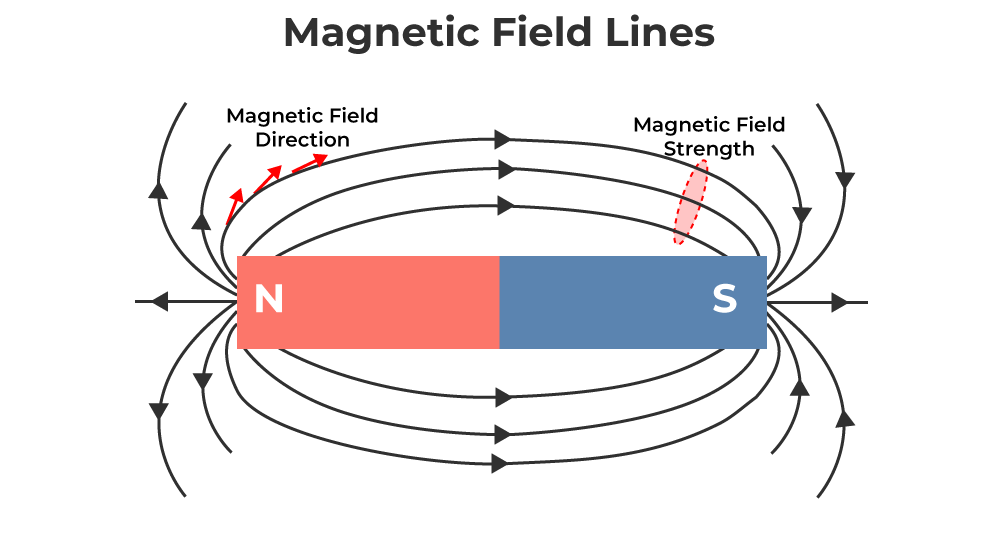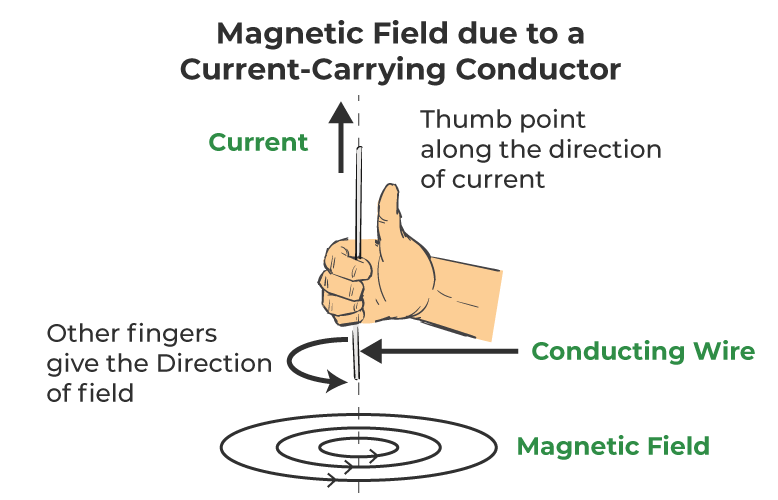GFG App
Open AppBrowser
Continue

# Magnetic Field due to Current carrying Conductor

Magnetic field is considered a region where the force of magnetism is present. This magnetic force is generally created by a moving charge or magnetic substance. H. C. Oersted made the discovery that a current-carrying conductor creates a magnetic influence all around it in the early years of the 19th century. Although science and technology were not as advanced as they are now, it was already known from observations that the effect of lightning striking a ship may result in the compass needles malfunctioning, which would interfere with the navigation system. Let’s learn more about the magnetic field due to the current-carrying conductor in this article!

## What are Magnetic Field Lines?

Magnetic field lines are imaginary lines around the magnet, and they are continuous closed loops. The tangent to the field line at any given point indicates the direction of the total magnetic field at that instant.

Since the magnet is dipolar, the magnetic lines must be originated and also have an end. Therefore, it starts from the north pole and terminates at the south pole outside the bar magnet, and it moves from the south pole to the north pole inside the magnet.

The closeness of field lines shows the relative strength of the magnetic field, i.e. closer lines show a stronger magnetic field and vice—versa. Crowded field lines near the poles of the magnet show more strength.Some important properties of magnetic field lines are:

• Magnetic field boundaries are never crossed.
• The depth of the field lines shows the field’s power.
• Magnetic field lines are often closed loops.
• Magnetic field lines often originate from or begin at the north pole and end at the South Pole.

## Magnetic Field Due to Current Carrying Conductor

When current is passed through a straight current-carrying conductor, a magnetic field is produced around it. The field lines are in the form of concentric circles at every point of the current-carrying conductor. And we can find the direction of the magnetic field, in relation to the direction of electric current through a straight conductor can be depicted by using the Right-Hand Thumb Rule also called as Maxwell Corkscrew Rule.This rule states that ‘If a current-carrying conductor is held by the right hand, keeping the thumb straight and if the direction of electric current is in the direction of thumb, then the direction of wrapping of other fingers will show the direction of the magnetic field.’

### Magnetic field due to current through a circular loop

The right-hand thumb rule can be used for a circular conducting wire as well as it comprises small straight segments. Every point on the wire carrying current gives rise to a magnetic field around it would become larger and larger as we move away from the wire and by the time we reach the center of the circular loop, the arcs of these circles would appear as a straight line

### Magnetic field and number of turns of the coil

The magnitude of the magnetic field gets summed up with the increase in the number of turns of the coil. If there are ‘n’ turns of the coil, the magnitude of the magnetic field will be ‘n’ times the magnetic field in case of a single turn of the coil.

The strength of the magnetic field at the center of the loop (coil) depends on:

• The radius of the coil: The strength of the magnetic field is inversely proportional to the radius of the coil. If the radius increases, the magnetic strength at the center decreases
• The number of turns in the coil: As the number of turns in the coil increase, the magnetic strength at the center increases, because the current in each circular turn is having the same direction, thus, the field due to each turn adds up.
• The strength of the current flowing in the coil: As the strength of the current increases, the strength of three magnetic fields also increases.

## Characteristics of Magnetic Field Due To Current Carrying Conductor

Following are the characteristics of the Magnetic Field due to Current Carrying Conductor:

• The lines of force near the wire are almost concentric circles.
• Moving toward the center of the loop, the concentric circles become larger and larger.
• Near the center of the loop, the arcs of these big circles appear as parallel straight lines.
• The magnetic field is almost uniform at the center of the loop.
• The magnitude of the magnetic field produced by a current-carrying circular loop (or circular wire) at its center is:
• Directly proportional to the current passing through the circular loop (or circular wire), and
• Inversely proportional to the radius of the circular loop (or circular wire).

## FAQs on Magnetic Field due to Current Carrying Conductor

Question 1: Explain the effect on the magnetic field produced at a point in a current-carrying circular coil due to an increase in the amount of current flowing through it.

The magnetic field produced by current carrying circular coil is directly proportional to the current flowing through the coil. Therefore, with increase in the magnitude of magnetic field the current flowing through the coil will increase.

Question 2: How does a Solenoid behave like a Magnet?

Since solenoid has iron core with insulated copper wire around it, therefore it behaves like magnet. When a current is flowing through the solenoid, magnetic field is produced around it. And the field produced is similar to the magnetic field of a bar magnet.

Question 3: Define the right-hand thumb rule.

This rule states that ‘If a current carrying conductor is held by right hand, keeping the thumb straight and if the direction of electric current is in the direction of thumb, then the direction of wrapping of other fingers will show the direction of magnetic field.’

Question 4: Why don’t two magnetic field lines cannot intersect each other?